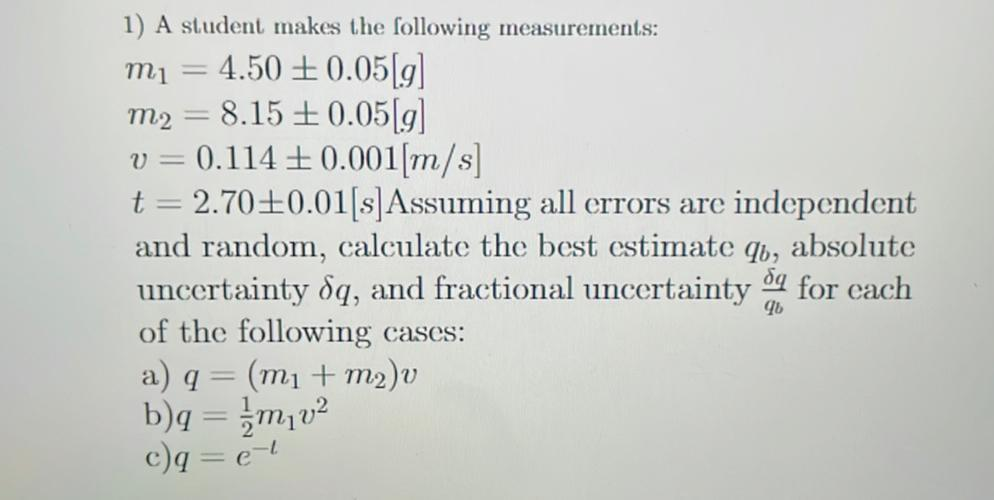Question:

# 1) A student makes the following measurements: mi 4.50 +0.0519) m2 = 8.15 + 0.05 g v = 0.114 +0.001[m/s] t = 2.70+0.01[s]Assumin1) A student makes the following measurements: mi 4.50 +0.0519) m2 = 8.15 + 0.05 g v = 0.114 +0.001[m/s] t = 2.70+0.01[s]Assuming all errors are independent and random, calculate the best estimate qb, absolute da uncertainty dq, and fractional uncertainty for cach of the following cases: a) q = (mi + m2)v b)q = {mv2 90 c)q = el